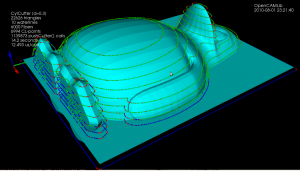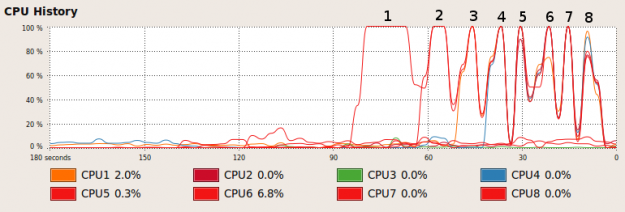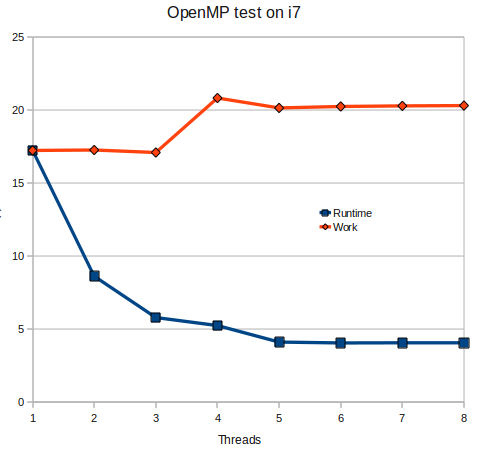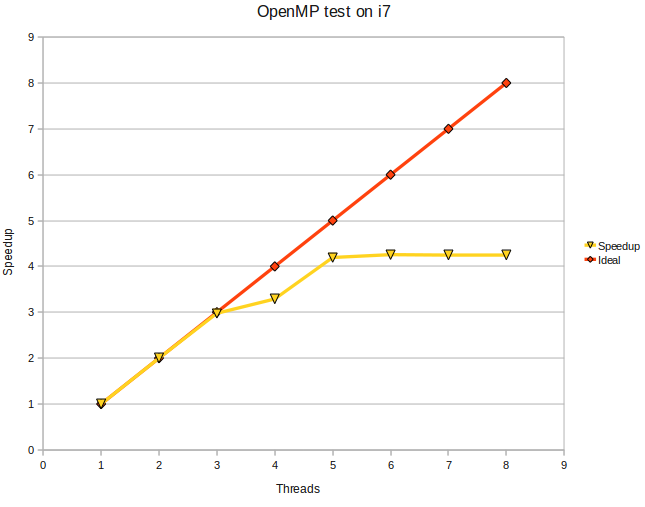## Faster waterlines with OpenMP

This example has three times more fibers, and thus also CL-points, than the original one, but it still runs in a reasonable time of ~15s because (1) I hard-coded the matrix-determinant expressions everywhere instead of relying on a slow general purpose function and (2) the batch-processing of the fibers now uses OpenMP in order to put all those multi-cores to work.

(red=vertex contacts, green=facet contacts, blue=edge contacts)

My initial point-ordering scheme based on a complete breadth-first-search at each CL-point is a bit naive and slow (that's not included in the 15s time), so that still needs more work.Over at Freesteel, Julian talks about using a "DiamondAngle" in the interval [0,4] for representing a plane angle instead of the usual radian in [0,2PI]. The argument is that using diangles there is an exact floating-point number for north/south/east/west, and that conversion to/from diangles is faster because it doesn't involve calling trigonometric functions.

I did a test with this, converting 10 million uniformly distributed radian angles to/from unit-vectors, and ditto for diangles. Converting diangles to unit-vectors is ca 25% faster than calling sin() and cos(), while the reverse transform is almost 3x faster than calling atan2. Runtimes in seconds below:

cos/sin 1.13
atan2 0.78
dia2vec 0.83
vec2dia 0.27
I will use a diangle to specify a position on the ellipse, for the offset-ellipse solver, which is central to the edge-drop function of drop-cutter for toroidal tools.

## Matrix determinant with Boost::uBLAS

Boost uBLAS provides BLAS functionality, but doesn't have a function for computing the determinant of a matrix. Googling for this turns up a few code snippets, but it's best to document this completely here now since I got it to work, and it will be useful for opencamlib sooner or later.

```1 2 3 4 5 6 7 8 9 10 11 12 13 14 15 16 17 18 19 20 21 22 23 24 25 26 27 28 29 30 31 32 33 34 35 36 37 38 39 40 41 42 #include <boost /numeric/ublas/matrix.hpp> #include </boost><boost /numeric/ublas/io.hpp> #include </boost><boost /numeric/ublas/lu.hpp>   namespace bnu = boost::numeric::ublas;   int determinant_sign(const bnu::permutation_matrix<std ::size_t>& pm) { int pm_sign=1; std::size_t size = pm.size(); for (std::size_t i = 0; i < size; ++i) if (i != pm(i)) pm_sign *= -1.0; // swap_rows would swap a pair of rows here, so we change sign return pm_sign; }   double determinant( bnu::matrix<double>& m ) { bnu::permutation_matrix</std><std ::size_t> pm(m.size1()); double det = 1.0; if( bnu::lu_factorize(m,pm) ) { det = 0.0; } else { for(int i = 0; i < m.size1(); i++) det *= m(i,i); // multiply by elements on diagonal det = det * determinant_sign( pm ); } return det; }   int main () { bnu::matrix<double> m(3, 3); for (unsigned i = 0; i < m.size1() ; ++i) { for (unsigned j = 0; j < m.size2() ; ++j) { m (i, j) = 3 * i + sqrt(j+1); // fill matrix m(i,j) = m(i,j)*m(i,j); // with some numbers } } std::cout << "before det() call m= " << m << std::endl; double det = determinant(m); std::cout << "after det() call m= " << m << std::endl; // m has changed afted determinant() call! std::cout << "determinant=" << det << std::endl; }```

I'm trying the WP-syntax plugin here for the first time. The include statements are garbled, but otherwise it seems to work.

This compiles on Lucid Lynx with the following CMakeLists.txt:

```cmake_minimum_required(VERSION 2.6)
PROJECT(utst1)
find_package( Boost )
if(Boost_FOUND)
include_directories(\${Boost_INCLUDE_DIRS})
endif()

## VTK test

Tried to make the code from last time a bit clearer by splitting it into two files:

conversion to video again by first converting PNG to JPEG:

mogrify -format jpg -quality 97 *.png

And then encoding JPEGs into a movie:

mencoder mf://*.jpg -mf fps=25:type=jpg -ovc lavc -lavcopts vcodec=mpeg4 -ac copy -o output.avi -ffourcc DX50

## OpenMP test on i7

Here's a simple piece of c-code (try zipped version) for testing how to parallelize code with OpenMP. It compiles with
`gcc -fopenmp -lm otest.c`

The CPU-load while running looks like this:Looks like two logical CPUs never get used (two low lines beyond "5" in the chart). It outputs some timing information:
``` running with 1 threads: runtime = 17.236827 s clock=17.230000 running with 2 threads: runtime = 8.624231 s clock=17.260000 running with 3 threads: runtime = 5.791805 s clock=17.090000 running with 4 threads: runtime = 5.241023 s clock=20.820000 running with 5 threads: runtime = 4.107738 s clock=20.139999 running with 6 threads: runtime = 4.045839 s clock=20.240000 running with 7 threads: runtime = 4.056122 s clock=20.280001 running with 8 threads: runtime = 4.062750 s clock=20.299999 ```
which can be plotted like this:I'm measuring the clock-cycles spent by the program using clock(), which I hope is some kind of measure of how much work is performed. Note how the amount of work increases due to overheads related to creating threads and communication between them. Another plot shows the speedup:The i7 uses Hyper Threading to present 8 logical CPUs to the system with only 4 physical cores. Anyone care to run this on a real 8-core machine ? 🙂

Next stop is getting this to work from a Boost Python extension.

## More pystones with shedskin

As I'm very much an amateur programmer with not too much time to learn new stuff I've decided my CAM-algorithms are going to be written in Python (don't hold your breath, they'll be online when they'll be online...). The benefits of rapid development will more than outweigh the performance issues of Python at this stage.

But then I found Mark Dufour's project shedskin (see also blog here and Mark's MSc thesis here), a Python to C++ compiler! Can you have the best of both worlds? Develop and debug your code interactively with Python and then, when you're happy with it, translate it automagically over to C++ and have it run as fast as native code?

Well, shedskin doesn't support any and all python constructs (yet?), and only a limited number of modules from the standard library are supported. But still I think it's a pretty cool tool. For someone who doesn't look forward to learning C++ from the ground up typing '`shedskin -e myprog.py`' followed by '`make`' is just a very simple way to create fast python extensions! As a test, I ran shedskin on the pystone benchmark and called both the python and c++ version from my multiprocessing test-code:

Python version

```Processes	Pystones	Wall time	pystones/s	Speedup
1		50000		0.7		76171		1.0X
2		100000		0.7		143808		1.9X
3		150000		0.7		208695		2.7X
4		200000		0.8		264410		3.5X
5		250000		1.0		244635		3.2X
6		300000		1.2		259643		3.4X
```

'shedskinned' C++ version

```Processes	Pystones	Wall time		pystones/s	Speedup
1		5000000			2.9		1696625		1.0X
2		10000000		3.1		3234625		1.9X
3		15000000		3.1		4901829		2.9X
4		20000000		3.4		5968676		3.5X
5		25000000		4.4		5714151		3.4X
6		30000000		5.1		5890737		3.5X
```

A speedup of around 20x.

## multiprocessing pystone benchmark

A simple pystone benchmark using the python multiprocessing package. Seems to scale quite well - guess how many cores my machine has! 🙂

``````
" Simple multiprocessing test.pystones benchmark "
" Anders Wallin 2008Jun15 anders.e.e.wallin (at) gmail.com "
from test import pystone
import processing
import time

STONES_PER_PROCESS= 10*pystone.LOOPS

def f(q):
t=pystone.pystones(STONES_PER_PROCESS)
q.put(t,block=True)

if __name__ == '__main__':
print 'multiprocessing test.pystones() benchmark'
print 'You have '+str(processing.cpuCount()) + ' CPU(s)'
print 'Processes\tPystones\tWall time\tpystones/s'

results = processing.Queue()
for N in range(1,processing.cpuCount()+3):
p=[]
q=processing.Queue()
results=[]

for m in range(1,N+1):
p.append( processing.Process(target=f,args=(q,)) )

start=time.time()
for pr in p:
pr.start()
for r in p:
results.append( q.get() )
stop=time.time()

cputime = stop-start

print str(N)+'\t\t'+str(N*STONES_PER_PROCESS) \
+'\t\t'+ str(cputime)+'\t'+str( N*STONES_PER_PROCESS / cputime )
``````